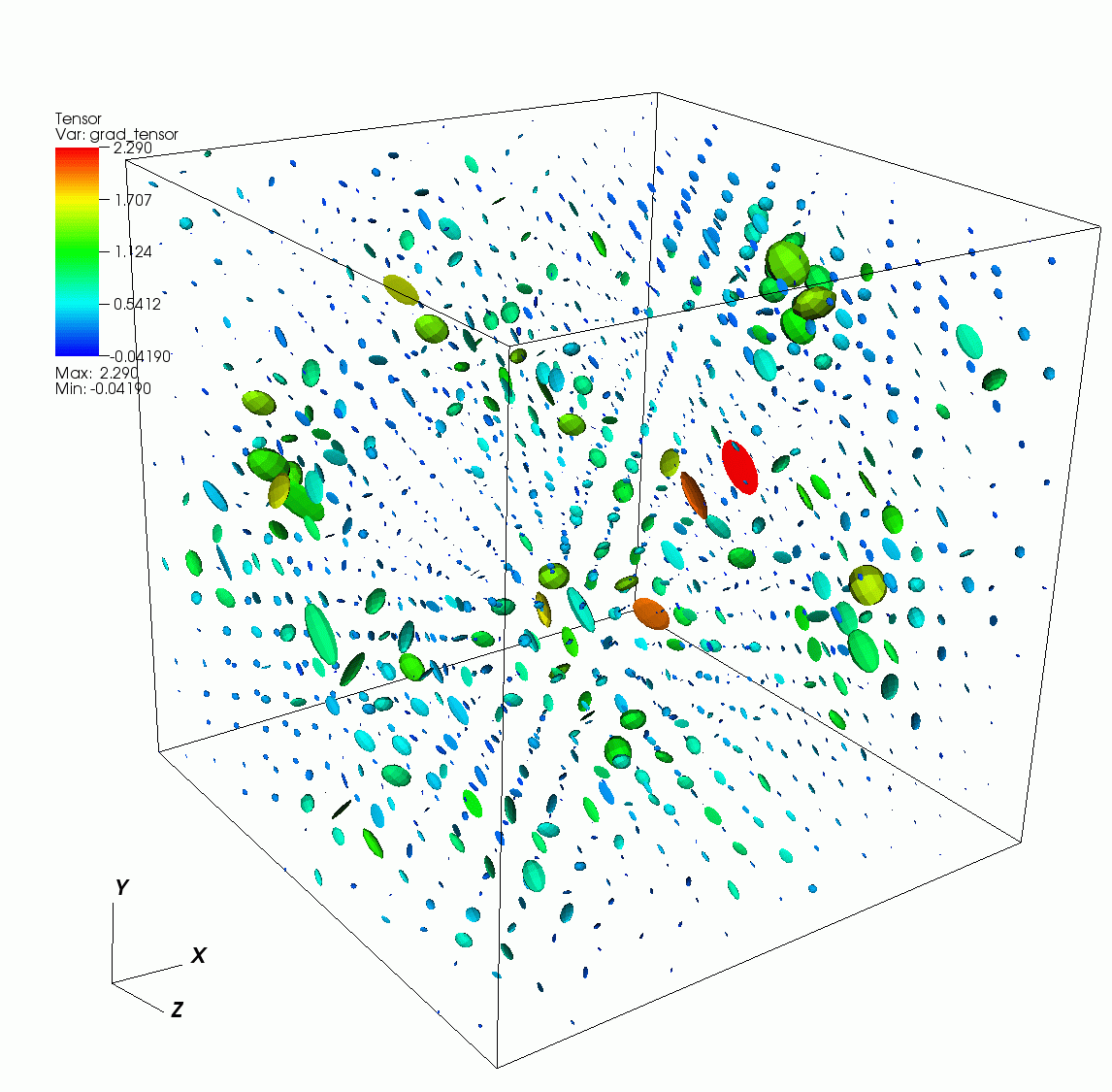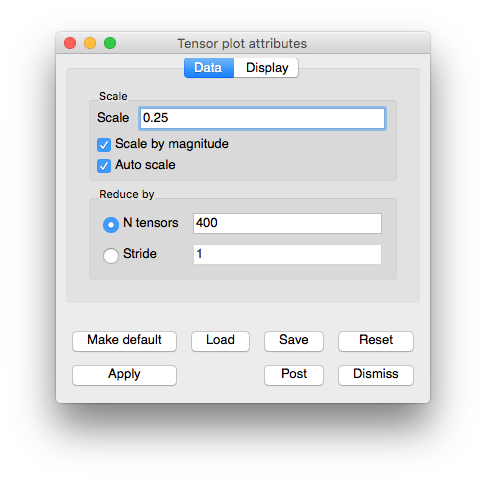# 3.2.12. Tensor plot¶

The Tensor plot, shown in Figure 3.50, displays tensor variables using ellipsoid glyphs to convey information about a tensor variable’s eigenvalues. Each glyph’s scaling and rotation is controlled by the eigenvalues/eigenvectors of the tensor as follows: for each tensor, the eigenvalues (and associated eigenvectors) are sorted to determine the major, medium, and minor eigenvalues/eigenvectors. The major eigenvalue scales the glyph in the x-direction, the medium in the y-direction, and the minor in the z-direction. Then, the glyph is rotated so that the glyph’s local x-axis lies along the major eigenvector, y-axis along the medium eigenvector, and z-axis along the minor.Fig. 3.50 Example of Tensor plotFig. 3.51 Tensor plot attributes window

## 3.2.12.1. Changing the tensor colors¶

The Tensor plot can be colored by a solid color or by the corresponding to the largest eigenvalue. To color the Tensor plot by eigenvalues, click the Eigenvalues radio button and then select a color table name from the color table button to the right of the Eigenvalues radio button. To make all tensor glyphs be the same color, click the Constant radio button and choose a color by clicking on the Constant color button and selecting a new color from the Popup color menu.

## 3.2.12.2. Setting the tensor scale¶

The Tensor plot’s tensor scale affects how large the ellipsoidal glyphs that represent the tensor are drawn. By default, VisIt computes an automatic scale factor based on the length of the bounding box’s diagonal to multiply by the user-specified scale factor. This ensures that the tensors are some reasonable size independent of the size of the mesh. To change the tensor scale, type a new floating point number into the Scale text field and click the Apply button in the Tensor plot attributes window. If you want to turn off automatic scaling so the size of the tensors is solely determined by the scale in the Scale text field, turn off the Auto scale check box. Yet another scaling option for tensors is scaling by magnitude. When the Scale by magnitude check box is checked, the magnitude of the tensor’s longest eigenvector is used as a scale factor that is multiplied into the scale determined by the user-specified scale and the automatic scale factor.

## 3.2.12.3. Setting the number of tensors¶

When visualizing a large database, a Tensor plot will often have too many tensors to effectively visualize so the Tensor plot provides controls to reduce the number of tensors to a number that looks appealing in a visualization. You can accomplish this reduction by setting a fixed number of tensors or by setting a stride. To set a fixed number of tensors, select the N tensors radio button and enter a new number of tensors into the N tensors text field. To reduce the number of tensors by setting the stride, select the Stride radio button and enter a new stride value into the Stride text field.